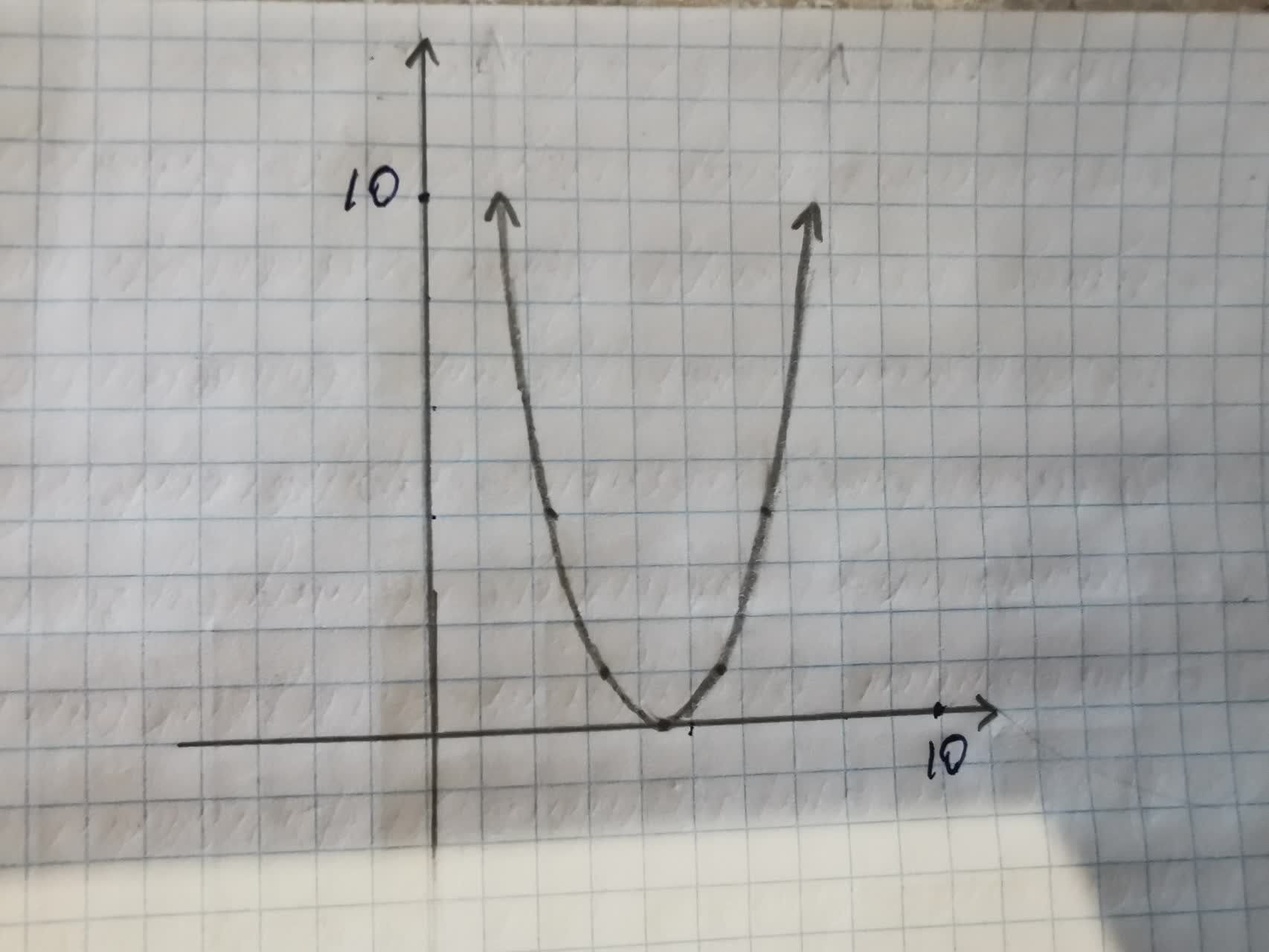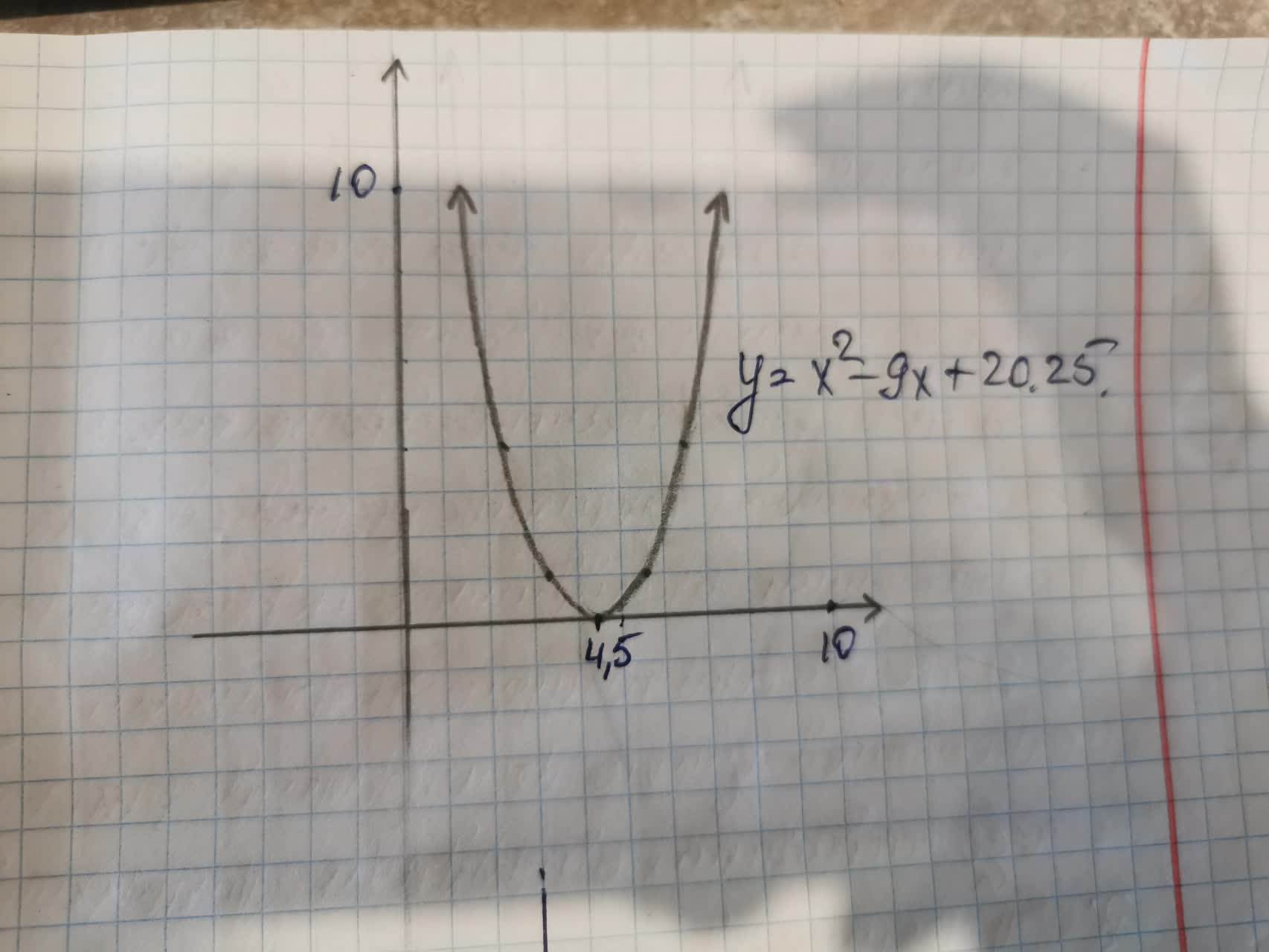# State the solutions, or estimated solutions, to the quadratic equationAnonym 2021-08-15 Answered
State the solutions, or estimated solutions, to the quadratic equation by examining the graph of the related quadratic function.
Equation:${x}^{2}-9x+20.25=0$
Graph:$y={x}^{2}-9x+20.25$You can still ask an expert for help

• Questions are typically answered in as fast as 30 minutes

Solve your problem for the price of one coffee

• Math expert for every subject
• Pay only if we can solve itirwchh
1. Find the solutions, or estimated solutions, if they exist, of the quadratic equation ${x}^{2}-9x+20.25=0$ by graphing $y={x}^{2}-9x+20.25$.
Use zoom tool, if neccessary, to find the points where the graph crosses the x-axis, where y equals 0- this will be the solution(s).2. The solutions to the equation ${x}^{2}-9x+20.25=0$ occur where the graph crosses the x-axis, at the point the y value is 0.
The graph crosses the x-axis at the points(4.5,0). The only solution is x=4.5
3. Check that 4.5 is a solution. Replace 4.5 for x in the equation ${x}^{2}-9x+20.25=0$
${x}^{2}-9x+20.25={\left(4.5\right)}^{2}-9\left(4.5\right)+20.25$
=20.25-40.5+20.25=0
This is a solution
###### Not exactly what you’re looking for?Jeffrey Jordon